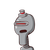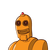# A linear calculation in two variations ax +by +c = 0 has, a) Infinity many solution b) a unique solution

A linear calculation in two variations ax +by +c = 0 has,

a) Infinity many solution
b) a unique solution
c) Two solutions
d) no solution​

### 2 thoughts on “A linear calculation in two variations ax +by +c = 0 has,<br /><br /> a) Infinity many solution<br /> b) a unique solution”

1.c )two solutions.

2.